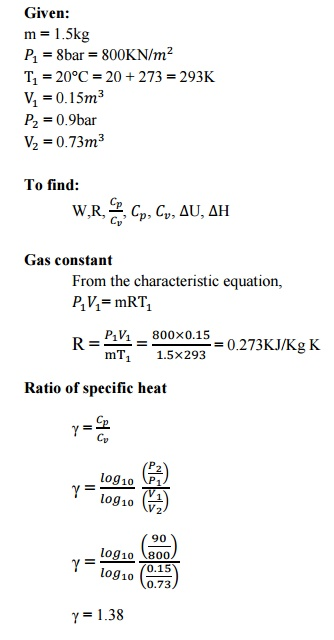Home | | Engineering Thermodynamics | Solved Problems: Basic Concepts and Thermodynamics First Law

# Solved Problems: Basic Concepts and Thermodynamics First Law

Mechanical - Engineering Thermodynamics - Basic Concepts And Definitions

1.A turbine operating under steady flow conditions receives steam at the following state: Pressure 13.8bar; Specific volume 0.143 Internal energy 2590 KJ/Kg; Velocity 30m/s. The state of the steam leaving the turbine is: Pressure 0.35bar; Specific Volume 4.37 Internal energy 2360KJ/Kg; Velocity 90m/s. Heat is lost to the surroundings at the rate of 0.25KJ/s. If the rate of steam flow is 0.38Kg/. What is the power developed by the turbine.2.Derive the steady flow energy equation and reduce it for a turbine, pump, nozzle and a heat exchanger.3.      0.25kg of air at a pressure of 1 bar occupies a volume of 0.3 . If this air expands isothermally to a volume of 0.9 . Find

i.                                                       The initial temperature

ii.                                                     Final temperature

iii.                                                  External work done

iv.                                                  Heat absorbed by the air

Change in internal energy. Assume R=0.29 KJ/Kg K.1.5kg of certain gas at a pressure of 8 bar and 20°C occupies the volume of 0.5 . It expands adiabatically to a pressure of 0.9 bar and volume 0.73 . Determine the work done during the process. Gas constant, ratio of specific heat, values of two specific heats, change in internal energy and change in enthalpy.5.                 A cylinder contains 1 of gas at 100kpa and 100°C, the gas is polytropically compressed to a volume of 0.25 . The final pressure is 100kpa. Determine

a.                                         Mass of the gas

b.                                        The   value   of   index   ‘n’   for   compression

c.                                          Change in internal energy of the gas

d.                                        Heat transferred by the gas during compression. Assume R = 0.287KJ/Kg K; γ= 1.4.Study Material, Lecturing Notes, Assignment, Reference, Wiki description explanation, brief detail
Mechanical : Engineering Thermodynamics : Basic Concepts And Definitions : Solved Problems: Basic Concepts and Thermodynamics First Law |